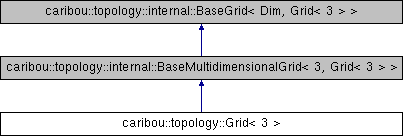Caribou
caribou::topology::Grid< 3 > Struct Reference
Inheritance diagram for caribou::topology::Grid< 3 >:## Public Types

using Base = internal::BaseMultidimensionalGrid< Dimension, Grid< Dimension > >

using FaceIndex = typename Base::UInt

using Element = geometry::RectangularHexahedron< Linear >

using Face = geometry::traits< Element >::BoundaryElementType

using Edge = geometry::traits< Face >::BoundaryElementType

using ElementNodes = std::array< NodeIndex, geometry::traits< Element >::NumberOfNodesAtCompileTime >

using FaceNodes = std::array< NodeIndex, geometry::traits< Face >::NumberOfNodesAtCompileTime >

using EdgeNodes = std::array< NodeIndex, geometry::traits< Edge >::NumberOfNodesAtCompileTime >

## Public Member Functions

auto cell_at (const GridCoordinates &coordinates) const noexcept -> Element

auto cell_at (const CellIndex &cell_index) const noexcept -> Element

auto node_indices_of (const GridCoordinates &cell_coordinates) const noexcept -> ElementNodes

auto node_indices_of (const CellIndex &index) const noexcept -> ElementNodes

auto number_of_edges () const noexcept -> UInt
Get the number of distinct edges in this grid.

auto edge (const EdgeIndex &index) const noexcept -> EdgeNodes
Get the node indices of the edge at edge index.

auto number_of_faces () const noexcept -> UInt
Get the number of distinct faces in this grid.

auto face (const FaceIndex &index) const noexcept -> FaceNodes
Get the node indices of the face at the given face index.

## Static Public Attributes

static constexpr size_t Dimension = 3

The documentation for this struct was generated from the following file:
• /home/jnbrunet/sources/caribou/src/Topology/Grid/Grid.h